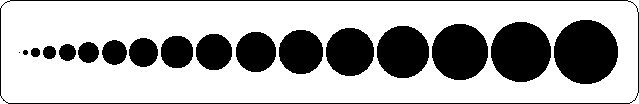# pldot

## draw a point (zero length line)

parametertypeunitsdescription
x,yfloatuuposition where the point is drawn
diameterfloatmmdiameter of the dot
returns:void

## Description

pldot draws a point (that is: a zero length line) with diameter diameter in the position (x,y) user units. Normally, plotting a zero length line would not do any actual plotting, but pldot forces the pen down on the paper.

## Examples

The following program plots a series of dots of increasing size:
```#include <simplot.h>
int main() {
float x=50;
int i;
plinit(PS,"pldot",A4,0,0,"","");
for (i=0;i<17;i++) {
x+=i+.5;
pldot(x,50,i);
}
plframe(5,3);
exit(0);
}
```pldotm

plreserv plloop plmvorg
• Home